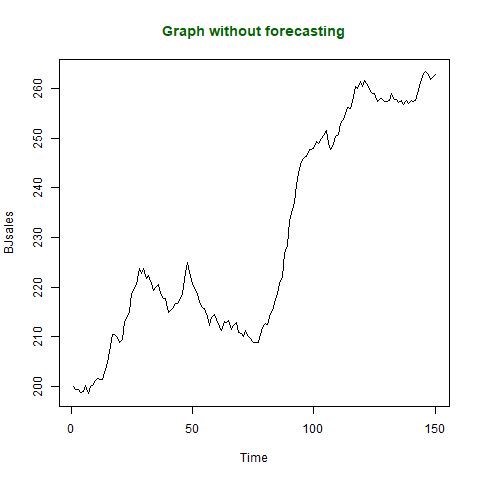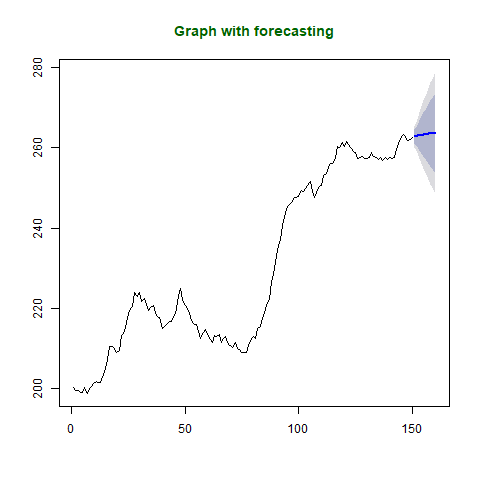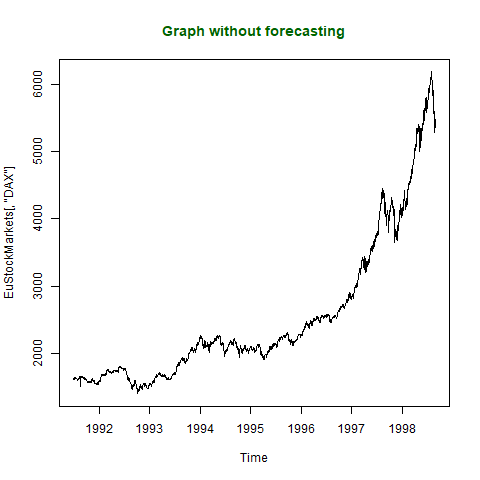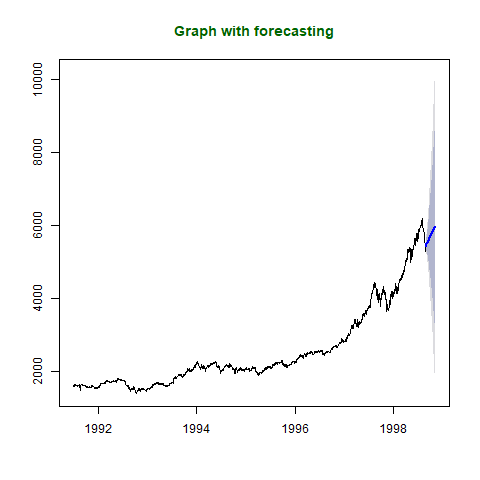# Time Series Analysis using ARIMA model in R Programming

In R programming, data analysis and visualization is so easy to learn the behaviour of the data. Moreover, the R language is used mostly in the data science field after Python. Time series analysis is a type of analysis of data used to check the behaviour of data over a period of time. The data is collected over time sequentially by the `ts()` function along with some parameters. It helps in analyzing the pattern of the data over a graph. There are many techniques used to forecast the time series object over the plot graph but the ARIMA model is the most widely used approach out of them.

#### Time Series Forecasting

Time series forecasting is a process of predicting future values with the help of some statistical tools and methods used on a data set with historical data. Some of the applications of time series forecasting are:

• Predicting stock prices
• Forecast weather
• Forecast the sales of a product

#### ARIMA model

ARIMA stands for AutoRegressive Integrated Moving Average and is specified by three order parameters: (p, d, q).

• AR(p) Autoregression: A regression model that utilizes the dependent relationship between a current observation and observations over a previous period.An auto regressive (AR(p)) component refers to the use of past values in the regression equation for the time series.
• I(d) Integration: Uses differencing of observations (subtracting an observation from observation at the previous time step) in order to make the time series stationary. Differencing involves the subtraction of the current values of a series with its previous values d number of times.
• MA(q) Moving Average: A model that uses the dependency between an observation and a residual error from a moving average model applied to lagged observations. A moving average component depicts the error of the model as a combination of previous error terms. The order q represents the number of terms to be included in the model.

Types of ARIMA Model

• ARIMA: Non-seasonal Autoregressive Integrated Moving Averages
• SARIMA: Seasonal ARIMA
• SARIMAX: Seasonal ARIMA with exogenous variables

#### Implementation of ARIMA model in R

In R programming, `arima()` function is used to perform this technique. ARIMA model is used to fit a univariate data. `auto.arima()` function returns the best ARIMA model by searching over many models.

Syntax:
auto.arima(x)

Parameters:
x: represents univariate time series object

To know about more optional parameters, use below command in the console: help(“auto.arima”)

Example 1:
In this example, let’s predict the next 10 sale values by using BJsales dataset present in R packages. This dataset is already a time series object, so there is no need to apply `ts()` function.

 `# R program to illustrate ` `# Time Series Analysis  ` `# Using ARIMA model in R ` ` `  `# Install the library for forecast() ` `install.packages``(``"forecast"``) ` ` `  `# library required for forecasting  ` `library``(forecast) ` ` `  `# Output to be created as png file ` `png``(file = ``"TimeSeriesGFG.png"``) ` ` `  `# Plotting graph without forecasting ` `plot``(BJsales, main = ``"Graph without forecasting"``, ` `col.main = ``"darkgreen"``) ` ` `  `# Saving the file ` `dev.off``() ` ` `  `# Output to be created as png file  ` `png``(file = ``"TimeSeriesARIMAGFG.png"``) ` ` `  `# Fitting model using arima model  ` `fit <- ``auto.arima``(BJsales) ` ` `  `# Next 10 forecasted values  ` `forecastedValues <- ``forecast``(fit, 10) ` ` `  `# Print forecasted values ` `print``(forecastedValues) ` ` `  `plot``(forecastedValues, main = ``"Graph with forecasting"``, ` `col.main = ``"darkgreen"``)  ` `    `  `# saving the file   ` `dev.off``()  `

Output:

```Point    Forecast   Lo 80    Hi 80    Lo 95    Hi 95
151       262.8620 261.1427 264.5814 260.2325 265.4915
152       263.0046 260.2677 265.7415 258.8189 267.1903
153       263.1301 259.4297 266.8304 257.4709 268.7893
154       263.2405 258.5953 267.8857 256.1363 270.3447
155       263.3377 257.7600 268.9153 254.8074 271.8680
156       263.4232 256.9253 269.9211 253.4855 273.3608
157       263.4984 256.0941 270.9028 252.1744 274.8224
158       263.5647 255.2691 271.8602 250.8778 276.2516
159       263.6229 254.4529 272.7930 249.5986 277.6473
160       263.6742 253.6474 273.7011 248.3395 279.0089
```Explanation:
Following output is produced by executing the above code. 10 next values are predicted by using `forecast()` function based on ARIMA model of BJsales dataset. First graph shows the visuals of BJsales without forecasting and second graphs shows the visuals of BJsales with forecasted values.

Example 2:
In this example, let’s predict next 50 values of DAX in EuStockMarkets dataset present in R base package. It can take longer time than usual as the dataset is large enough as compared to BJsales dataset. This dataset is already a time series object, so there is no need to apply `ts()` function.

 `# R program to illustrate ` `# Time Series Analysis  ` `# Using ARIMA model in R ` ` `  `# Install the library for forecast() ` `install.packages``(``"forecast"``) ` ` `  `# library required for forecasting  ` `library``(forecast) ` ` `  `# Output to be created as png file ` `png``(file = ``"TimeSeries2GFG.png"``) ` ` `  `# Plotting graph without forecasting ` `plot``(EuStockMarkets[, ``"DAX"``], ` `main = ``"Graph without forecasting"``, ` `col.main = ``"darkgreen"``) ` ` `  `# Saving the file ` `dev.off``() ` ` `  `# Output to be created as png file  ` `png``(file = ``"TimeSeriesARIMA2GFG.png"``) ` ` `  `# Fitting model using arima model  ` `fit <- ``auto.arima``(EuStockMarkets[, ``"DAX"``]) ` ` `  `# Next 50 forecasted values  ` `forecastedValues <- ``forecast``(fit, 50) ` ` `  `# Print forecasted values ` `print``(forecastedValues) ` ` `  `plot``(forecastedValues, main = ``"Graph with forecasting"``, ` `col.main = ``"darkgreen"``)  ` `    `  `# saving the file   ` `dev.off``()  `

Output:

``` Point         Forecast    Lo 80    Hi 80    Lo 95     Hi 95
1998.650       5477.071 5432.030 5522.113 5408.186  5545.957
1998.654       5455.437 5385.726 5525.148 5348.823  5562.051
1998.658       5438.930 5345.840 5532.020 5296.561  5581.299
1998.662       5470.902 5353.454 5588.349 5291.281  5650.522
1998.665       5478.529 5334.560 5622.498 5258.347  5698.710
1998.669       5505.691 5333.182 5678.199 5241.862  5769.519
1998.673       5512.314 5306.115 5718.513 5196.960  5827.669
1998.677       5517.260 5276.482 5758.037 5149.022  5885.497
1998.681       5524.894 5248.363 5801.426 5101.976  5947.813
1998.685       5540.523 5226.767 5854.279 5060.675  6020.371
1998.688       5551.386 5198.746 5904.026 5012.070  6090.702
1998.692       5564.652 5171.572 5957.732 4963.488  6165.815
1998.696       5574.519 5139.172 6009.867 4908.713  6240.326
1998.700       5584.631 5105.761 6063.500 4852.263  6316.998
1998.704       5595.439 5071.763 6119.116 4794.545  6396.333
1998.708       5607.468 5037.671 6177.265 4736.039  6478.897
1998.712       5618.547 5001.313 6235.781 4674.569  6562.524
1998.715       5629.916 4963.981 6295.852 4611.456  6648.377
1998.719       5640.767 4924.864 6356.669 4545.888  6735.645
1998.723       5651.753 4884.718 6418.789 4478.674  6824.833
1998.727       5662.881 4843.558 6482.203 4409.835  6915.926
1998.731       5674.177 4801.426 6546.928 4339.419  7008.934
1998.735       5685.286 4757.984 6612.588 4267.100  7103.472
1998.738       5696.434 4713.486 6679.383 4193.144  7199.725
1998.742       5707.511 4667.838 6747.183 4117.468  7297.553
1998.746       5718.625 4621.180 6816.069 4040.228  7397.022
1998.750       5729.763 4573.514 6886.012 3961.433  7498.093
1998.754       5740.921 4524.851 6956.990 3881.103  7600.739
1998.758       5752.044 4475.153 7028.934 3799.208  7704.879
1998.762       5763.173 4424.479 7101.867 3715.817  7810.528
1998.765       5774.293 4372.828 7175.758 3630.938  7917.649
1998.769       5785.422 4320.235 7250.610 3544.611  8026.233
1998.773       5796.554 4266.706 7326.403 3456.853  8136.256
1998.777       5807.688 4212.254 7403.123 3367.682  8247.695
1998.781       5818.816 4156.883 7480.749 3277.109  8360.523
1998.785       5829.945 4100.614 7559.276 3185.162  8474.729
1998.788       5841.073 4043.457 7638.690 3091.856  8590.291
1998.792       5852.203 3985.425 7718.982 2997.212  8707.195
1998.796       5863.333 3926.528 7800.139 2901.245  8825.422
1998.800       5874.464 3866.776 7882.152 2803.970  8944.957
1998.804       5885.593 3806.178 7965.007 2705.402  9065.783
1998.808       5896.722 3744.746 8048.698 2605.559  9187.886
1998.812       5907.852 3682.489 8133.214 2504.453  9311.250
1998.815       5918.981 3619.416 8218.547 2402.100  9435.863
1998.819       5930.111 3555.536 8304.686 2298.512  9561.710
1998.823       5941.241 3490.857 8391.624 2193.702  9688.779
1998.827       5952.370 3425.388 8479.352 2087.684  9817.056
1998.831       5963.500 3359.137 8567.862 1980.470  9946.529
1998.835       5974.629 3292.111 8657.147 1872.072 10077.186
1998.838       5985.759 3224.319 8747.198 1762.502 10209.016
```Explanation:
The following output is produced by executing the above code. 50 future stock price of DAX is predicted by using `forecast()` function based on the ARIMA model of DAX of EuStockMarkets dataset. The first graph shows the visuals of DAX of EuStockMarkets dataset without forecasting and second graphs show the visuals of DAX of EuStockMarkets dataset with forecasted values.

My Personal Notes arrow_drop_upCheck out this Author's contributed articles.

If you like GeeksforGeeks and would like to contribute, you can also write an article using contribute.geeksforgeeks.org or mail your article to contribute@geeksforgeeks.org. See your article appearing on the GeeksforGeeks main page and help other Geeks.

Please Improve this article if you find anything incorrect by clicking on the "Improve Article" button below.

Article Tags :

1

Please write to us at contribute@geeksforgeeks.org to report any issue with the above content.